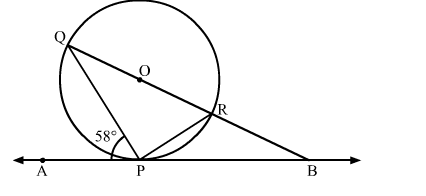# In the given figure, O is the centre of the circle. AB is the tangent to the circle at the point P.`
Question:

In the given figure, O is the centre of the circle. AB is the tangent to the circle at the point P. If  ∠APQ = 58  then the measue of  ∠PQB is  [CBSE 2014]
(a) 32
(b) 58
(c) 122
(d) 132Solution:

We know that a chord passing through the centre is the diameter of the circle.
∵∠QPR = 90    (Angle in a semi circle is 90)
By using alternate segment theorem
We have ∠APQ = ∠PRQ = 58
Now, In △PQR
∠PQR + ∠PRQ + ∠QPR = 1800            [Angle sum property of a triangle]
⇒ ∠PQR + 58 +  900 = 180
⇒ ∠PQR= 32
Hence, the correct answer is option (a).